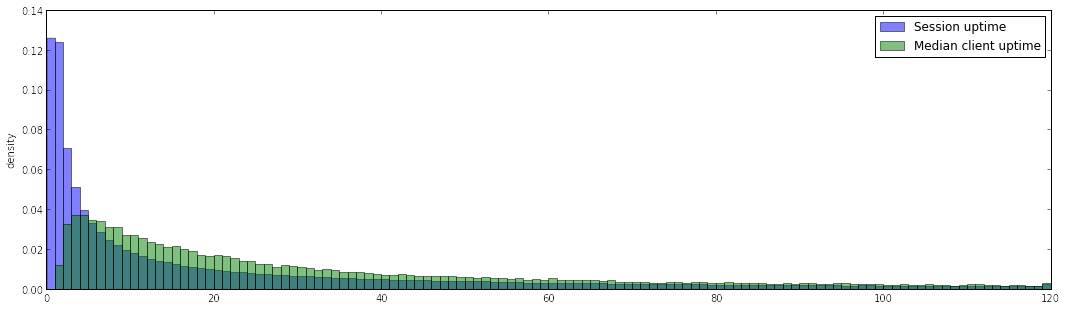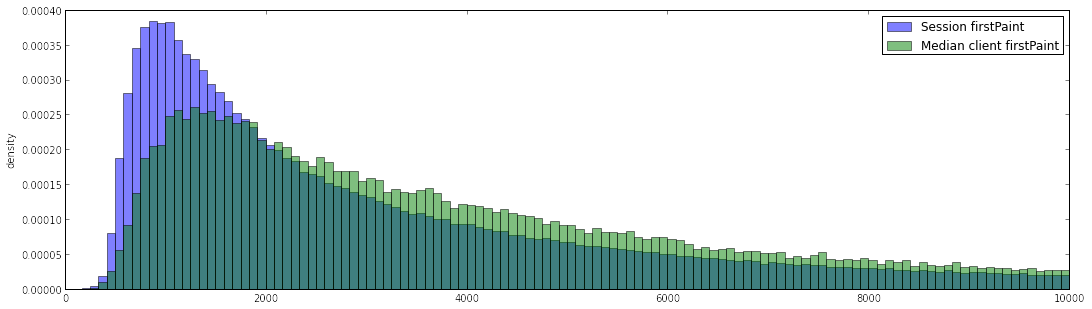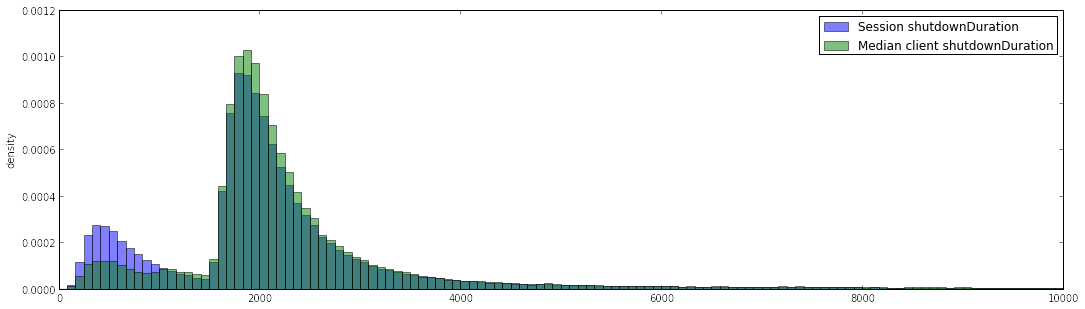### ClientID "Mode" EDA¶

In :
import simplejson as json
import pandas as pd
import numpy as np
import matplotlib.pyplot as plt

from moztelemetry.spark import get_pings
from __future__ import division
from operator import itemgetter

In :
%%capture

pings = get_pings(sc, "Firefox", "nightly", "37.0a1", "*", ("20141208", "20141214"))

In :
%%capture

def extract(ping):
info = ping["info"]
simple = ping["simpleMeasurements"]

ping_subset = dict(info.items() + simple.items())
ping_subset["clientID"] = ping.get("clientID", None)
ping_subset.pop("UITelemetry", None)

return ping_subset

pings_summary = pings.map(extract)

In :
%%capture
df = pd.DataFrame(pings_summary.collect())
df = df[df["uptime"] >= 0]

In :
table = pd.pivot_table(df, index="clientID", \
values=["uptime", "memsize", "cpucount", "firstPaint", "shutdownDuration", "failedProfileLockCount"], \
aggfunc=[len, np.median])
table = table.swaplevel(0, 1, axis=1).sortlevel(0, axis=1)


#### EDA¶

Let's compare the distributions of some metrics to see if we spot interesting differences. Given a metric, we are comparing the distribution of the median client measurement to the distribution of the metric in the sessions.

##### Summary Statistics for clients¶
In :
percentiles = [0.1, 0.25, 0.5, 0.75, 0.9]
table.swaplevel(0, 1, axis=1).sortlevel(0, axis=1)["median"].describe(percentiles=percentiles)

Out:
cpucount failedProfileLockCount firstPaint memsize shutdownDuration uptime
count 75620.000000 3279.000000 7.561900e+04 75620.000000 7.472100e+04 75620.000000
mean 4.129913 2.115584 1.815358e+04 6505.502975 2.290299e+04 845.903313
std 2.297999 5.006241 1.364399e+06 5722.442852 1.991885e+06 51529.107735
min 1.000000 1.000000 1.810000e+02 191.000000 3.500000e+01 1.000000
10% 2.000000 1.000000 1.168400e+03 2005.000000 1.322500e+03 5.000000
25% 2.000000 1.000000 1.892000e+03 3683.000000 1.782000e+03 12.000000
50% 4.000000 1.000000 3.629000e+03 4091.000000 2.046500e+03 35.000000
75% 4.000000 2.000000 7.080000e+03 8104.000000 2.553000e+03 129.000000
90% 8.000000 3.000000 1.383680e+04 15740.300000 4.009000e+03 531.000000
max 64.000000 141.000000 2.713677e+08 262134.000000 3.376794e+08 7331914.000000
##### Summary statistics for sessions¶
In :
df[table.columns.levels].describe(percentiles = percentiles)

Out:
cpucount failedProfileLockCount firstPaint memsize shutdownDuration uptime
count 947542.000000 4279.000000 9.471660e+05 947542.000000 7.827300e+05 947542.000000
mean 4.213679 2.214536 2.182672e+04 6688.532153 1.861458e+04 1033.239616
std 2.331205 4.976688 9.109550e+06 5688.972728 1.384553e+06 77354.866387
min 1.000000 1.000000 1.300000e+02 191.000000 2.400000e+01 0.000000
10% 2.000000 1.000000 8.590000e+02 2013.000000 6.200000e+02 0.000000
25% 2.000000 1.000000 1.350000e+03 3817.000000 1.704000e+03 2.000000
50% 4.000000 1.000000 2.702000e+03 4095.000000 1.999000e+03 15.000000
75% 4.000000 2.000000 6.134000e+03 8124.000000 2.531000e+03 80.000000
90% 8.000000 4.000000 1.426400e+04 16107.000000 4.135000e+03 313.000000
max 64.000000 141.000000 8.842326e+09 262134.000000 5.272277e+08 18376554.000000

Of the considered metrics the ones that exhibit a difference are uptime, firstPaint and shutdownDuration (in the lower quartile) and failedProfileLockCount (in the upper quartile).

### uptime¶

As the plot below shows, using the median session uptime for a client does increase significantly the perceived uptime duration, i.e. we are overrepresenting sessions with short uptimes when not aggregating by clients.

In :
def compare_distributions(metric, max_min):
stat = table[metric]["median"]

plt.figure(figsize=(18, 5))
plt.ylabel("density")
plt.hist(df[metric], 120, range=(0, max_min), normed=1, alpha=0.5, label="Session " + metric)
plt.hist(stat, 120, range=(0, max_min), normed=1, alpha=0.5, label="Median client " + metric)
plt.legend(loc='upper right')
plt.show()

compare_distributions("uptime", 120)#### firstPaint¶

The startup duration is considerably higher if we don't overcount submissions from the same users.

In :
compare_distributions("firstPaint", 10000)#### shutdownDuration¶

We are overcounting very short shutdown durations when not aggregating by client.

In :
compare_distributions("shutdownDuration", 10000)In [ ]: